### IMO Shortlist 1994 problem G4

Kvaliteta:
Avg: 0,0
Težina:
Avg: 6,5
Let$ABC$ be an isosceles triangle with$AB = AC$.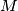$M$ is the midpoint of$BC$ and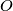$O$ is the point on the line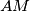$AM$ such that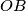$OB$ is perpendicular to$AB$.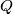$Q$ is an arbitrary point on$BC$ different from$B$ and$C$.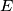$E$ lies on the line$AB$ and$F$ lies on the line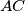$AC$ such that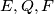$E, Q, F$ are distinct and collinear. Prove that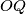$OQ$ is perpendicular to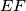$EF$ if and only if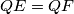$QE = QF$.
Izvor: Međunarodna matematička olimpijada, shortlist 1994